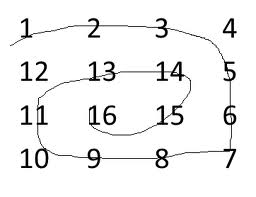# Python Program to Print matrix in antispiral form

• Last Updated : 06 Jan, 2022

Given a 2D array, the task is to print matrix in anti spiral form:
Examples:Output: 16 15 14 13 12 11 10 9 8 7 6 5 4 3 2 1

```Input : arr[] = {1, 2, 3, 4
5, 6, 7, 8
9, 10, 11, 12
13, 14, 15, 16};
Output : 10 11 7 6 5 9 13 14 15 16 12 8 4 3 2 1

Input :arr[] = {1, 2, 3, 4, 5, 6
7, 8, 9, 10, 11, 12
13, 14, 15, 16, 17, 18};
Output : 11 10 9 8 7 13 14 15 16 17 18 12 6 5 4 3 2 1```

The idea is simple, we traverse matrix in spiral form and put all traversed elements in a stack. Finally one by one elements from stack and print them.

## Python 3

 `# Python 3 program to print``# matrix in anti-spiral form``R ``=` `4``C ``=` `5`` ` `def` `antiSpiralTraversal(m, n, a):``    ``k ``=` `0``    ``l ``=` `0`` ` `    ``# k - starting row index``    ``# m - ending row index``    ``# l - starting column index``    ``# n - ending column index``    ``# i - iterator ``    ``stk ``=` `[]`` ` `    ``while` `(k <``=` `m ``and` `l <``=` `n):``         ` `        ``# Print the first row ``        ``# from the remaining rows ``        ``for` `i ``in` `range``(l, n ``+` `1``):``            ``stk.append(a[k][i])``        ``k ``+``=` `1`` ` `        ``# Print the last column ``        ``# from the remaining columns ``        ``for` `i ``in` `range``(k, m ``+` `1``):``            ``stk.append(a[i][n])``        ``n ``-``=` `1`` ` `        ``# Print the last row``        ``# from the remaining rows ``        ``if` `( k <``=` `m):``            ``for` `i ``in` `range``(n, l ``-` `1``, ``-``1``):``                ``stk.append(a[m][i])``            ``m ``-``=` `1`` ` `        ``# Print the first column ``        ``# from the remaining columns ``        ``if` `(l <``=` `n):``            ``for` `i ``in` `range``(m, k ``-` `1``, ``-``1``):``                ``stk.append(a[i][l])``            ``l ``+``=` `1``         ` `    ``while` `len``(stk) !``=` `0``:``        ``print``(``str``(stk[``-``1``]), end ``=` `" "``)``        ``stk.pop()`` ` `# Driver Code``mat ``=` `[[``1``, ``2``, ``3``, ``4``, ``5``],``       ``[``6``, ``7``, ``8``, ``9``, ``10``],``       ``[``11``, ``12``, ``13``, ``14``, ``15``],``       ``[``16``, ``17``, ``18``, ``19``, ``20``]];`` ` `antiSpiralTraversal(R ``-` `1``, C ``-` `1``, mat)`` ` `# This code is contributed``# by ChitraNayal`

Output:

`12 13 14 9 8 7 6 11 16 17 18 19 20 15 10 5 4 3 2 1 `

Please refer complete article on Print matrix in antispiral form for more details!

My Personal Notes arrow_drop_up